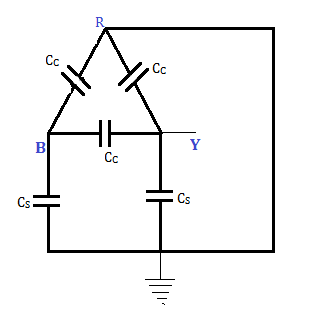# Grading of Cables & Capacitance of Three core cables - MCQs with Answers

## Grading of Cables & Capacitance of Three core cables - MCQs with Answers

Q1. What does capacitance grading of cables mean?

a. Use of dielectrics in different concentrations.
b. Introduction of capacitance at various lengths of cable to counter the effect of inductance.
c. Use of dielectrics of different permittivities.
d. Grading according to capacitance per km length of the cable.

ANSWER: c. Use of dielectrics of different permittivities.

Q2. In a 3 core cable, the capacitance between two conductors is 3 μF. What will be the capacitance per phase?

a. 1.5 μ F
b. 3 μ F
c. 6 μ F
d. 12 μ F

ANSWER: c. 6 μ F

Q3. For the fig shown, what will be the capacitance between A and Ba. CC + (CS / 2)
b. Cs + (Cc / 2)
c. (CS + 3 Cc) / 2
d. 3CC + 2CS

ANSWER: c. (CS + 3 Cc) / 2

Q4. What is the source of heat generation in cables?

a. Copper loss in conductor
b. Dielectric losses in cable insulation
c. Losses in metallic sheathings and armouring.
d. All of these.

ANSWER: d. All of these.

Q5. The capacitances of a 3 phase belted cable are 12.6 μ F between the three cores bunched together and the lead sheath and 7.4 μ F between one core and the other two connected to sheath. What will be the charging current drawn by the cable when connected to a 66 kV supply?

a. 100 A
b. 99.3648 A
c. 105.236 A
d. 107.74 A

ANSWER: d. 107.74 A

Q6. What is the safe working temperature for a conductor in case of armoured cables?

a. 50° C
b. 75° C
c. 65° C
d. 40° C

ANSWER: c. 65° C

Q7. Which tests are conducted to locate the fault location in the cables?

a. Murray loop test.
b. Varley loop test.
c. Both (a) and (b).
d. None of these.

ANSWER: c. Both (a) and (b).

Q8. In a test by Murray loop for ground fault on 500 m of cable having a resistance of 1.6 Ω / km, the faulty cable is looped with the sound cable of some length and area of cross section. If the ratio of the other two arms of the testing network at balance is 3:1, what is the distance of fault from the testing end of cables?

a. 100 m
b. 200 m
c. 250 m
d. 300 m